Strongly Correlated Photons in a Two-Dimensional Array of Photonic Crystal Microcavities

Y. C. Neil Na June 1, 2007

(Submitted as coursework for Applied Physics 273, Stanford University, Spring 2007)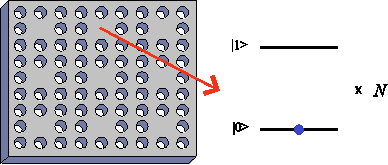Fig. 1: Schematic plot for a two-dimensional array of photonic crystal microcavities doped with substitutional donor/acceptor impurities.

Quantum many-body systems, such as strongly correlated electrons, are generally difficult to understand due to the lack of appropriate theoretical tools. A brute-force matrix diagonalization method is limited by the exponentially growing Hilbert space dimension with the number of particles. A quantum Monte-Carlo simulation method often suffers from the so-called sign problem. An analytical mean-field method provides good approximate solutions for three-dimensional systems but limited applications for two-dimensional systems. An interesting alternative is to construct a quantum simulator that implements a model Hamiltonian with controllable parameters . One such example was demonstrated using Bose-condensed cold atoms in an optical lattice potential. The superfluid to Mott-insulator QPT predicted by the Bose-Hubbard model was observed by changing the ratio of on-site repulsive interaction to hopping matrix element U/t . This experiment opened a door for simulating complex many-body systems with more controllable artificial systems [3,4].

Simulating the Bose-Hubbard model using photons has recently attracted an intense interest [5,6,7]. Even though photons do not interact with each other in free space, by confining light inside a small cavity with an active medium, the nonlinear photon-photon interaction can be effectively introduced. A related concept is a photon blockade: an optical analog of single electron Coulomb blockade effect [8,9]. These schemes require an extremely high-Q cavity even though EIT in a four-level atomic ensemble or single-atom cavity QED in the strong coupling regime are employed.

Here we show that an optical quantum simulator for such strongly correlated photonic systems can be constructed using a simpler approach. Our scheme consists of a two-dimensional array of coupled photonic crystal microcavities  doped with substitutional donor/acceptor impurities [11,12]. A schematic plot of the system is shown in Fig. 1. The photons hop from site to site via optical evanescent field coupling and interact with each other through the nonlinearity induced by the many-exciton cavity QED effect. Setting bulk doping density to 1014 cm-3, cavity photon-bound exciton frequency detuning to hundreds of GHz and cavity Q factor to 105, the QPT from photonic superfluid to Mott-insulator should be observed. The proposed scheme combines the large oscillator strength and small inhomogeneous linewidth of donor/acceptor-bound excitons embedded in bulk semiconductor matrix , and the recent advancement in photonic crystal microcavities with high cavity Q factor and small mode volume . In particular, we will show that when using a blue detuning, the increase of exciton fraction of the lower branch of the cavity polaritons decreases the required cavity Q factor.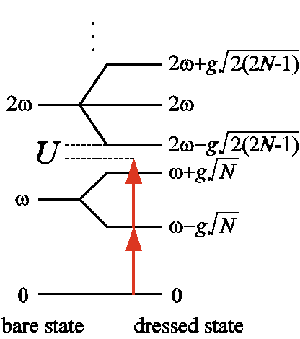Fig. 2: The eigenenergy spectrum of a many-exciton cavity QED system at zero frequency detuning. N = 2 is assumed. The ground state interaction energy U for adding a subsequent photon to the cavity is labeled.

We start our analysis by considering the optical evanescent field coupling between adjacent microcavities. The tunneling part of the Hamiltonian is given as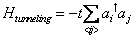where <ij> indicates that only the nearest neighbor coupling is considered, t is the tunneling energy determined by the overlapping of the nearest neighbor cavity fields, and ai is the annihilation operator of the ith site cavity mode. To quantitatively estimate the condition of QPT, we perform a mean field analysis by applying the decoupling approximation [7,14] i.e. let aiaj = < ai> aj + ai < aj> - < ai> < aj> and define a real-valued superfluid parameter ψ = < ai>. The Hamiltonian can then be rewritten as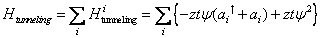where z is the number of nearest neighbors. Next we consider the free and interacting part of the total Hamiltonian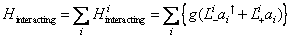ωe, ωp and g are the bound exciton transition frequency, cavity photon resonance frequency and exciton-photon coupling constant. N is the number of impurities per cavity. Lz is the collective angular momentum operator in the z-direction and L± are the collective creation/annihilation operators. The single site eigenenergy spectrum considering only the free and interacting Hamiltonian is sketched in Fig. 2. In general, the number of eigenstates for each excitation manifold n is equal to n+1 if n≤N and equal to N+1 if n>N. The ground state (lower branch of the cavity polaritons for n=1 excitation manifold) interaction energy U can then be identified and is shown in Fig. 2. Notice that in the small detuning limit, U approaches zero if N is much larger than n. In this case, the system behaves linearly because the collective angular momentum operator satisfies a bosonic commutation relation [L+, L-] ~ N .

Equipped with these equations, we are now in position to evaluate the QPT condition. We consider only a single site Hamiltonian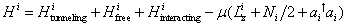where μ is the chemical potential in grand canonical ensemble. Given t and μ, one can calculate the eigenenergies by diagonalizing the effective Hamiltonian using bare states as a complete set of basis. The ground state energy can be found by minimizing the lowest eigenenergy with adjusting the superfluid parameter. The accuracy of such calculation depends on how many state vectors are used to span the Hilbert space. The convergence of the eigenenergies is usually a good indication that a large enough basis set is considered.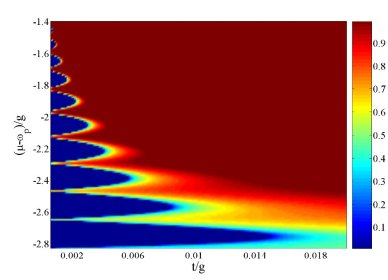Fig. 3: Zero-temperature phase diagram obtained by plotting the superfluid parameter as a function of t and μ. N = and Δ = 0 are assumed. The lowest Mott lobe corresponds to one photon per cavity, the second lowest Mott lobe corresponds to two photons per cavity and so forth.

In Fig. 3, we plot the superfluid parameter as a function of t and μ given N = 8 and Δ = ωp - ωe = 0. Unlike the single-atom cavity QED systems [6,7], the Mott lobe sizes in the chemical potential direction are relatively unchanged for low filling factors. The nature of such a photonic QPT is neither purely fermionic nor bosonic, but shares similar features with the Bose-Hubbard model: localization of one additional photon per cavity upon entering the next Mott-insulator regime.

To understand the general behavior of the QPT condition, we plot the required t for the system to reach the QPT as a function of N and Δ. This is shown in Fig. 4a. The numerical value of ωe/2π is chosen as 365.8 THz that corresponds to 820 nm Si donor-bound exciton emission wavelength . g is estimated as 33.2 GHz by calculating the bound exciton oscillator strength using the experimentally measured 1 ns lifetime , and a cavity mode volume equal to (820/3.25)3 nm3. (3.25 is the refractive index of GaAs.) As previously explained, the system behaves linearly if N is sufficiently large and hence t decreases as N increases. In addition, by having a blue detuning i.e. &Delta > 0, the ground state is of more exciton fraction. Therefore, t is enhanced because the optical evanescent field coupling has to be stronger in order to maintain the QPT condition.

The leakage of the cavity photons imposes an important constraint on the required cavity Q factor. If the polariton decay rate in the Mott-insulator regime is faster than the polariton tunneling rate, the system never reaches an equilibrium state described by Hamiltonian (5). Assuming that the ground state decay rate is slower than the tunneling rate, we find the required cavity Q factor to be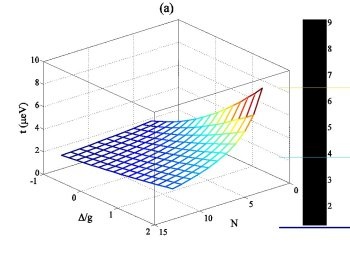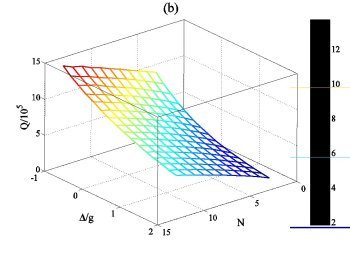Fig. 4: The required (a) tunneling energy and (b) cavity Q factor for the system to enter the Mott-insulator regime with one photon per cavity as a function of N and Δ.

where |ce2 and |cp|2 are the exciton and photon fraction of the ground state, t is the required tunneling energy for the system to reach the QPT, τe is the bound exciton spontaneous emission lifetime, and F is the Purcell factor due to the inhibition of spontaneous emission in a photonic crystal results from the reduced local optical density of states. Notice that the polariton tunneling rate is well described by |cp|2t. We plot the required Q value in Fig. 4b as a function of N and Δ where F = 0.2 is used [16,17]. In general, Q about 105 to 106 is needed to reach the OPT point. However, with a blue detuning, the cavity Q factor is reduced. This way, in principle, cavity Q factor can be relaxed by choosing a sufficiently large blue detuning. For example, Q is slightly smaller than 105 when Δ = 12 g given N = 9.

Observing the QPT from a photonic superfluid to Mott-insulator requires the ability to control t and U. The frequency detuning can be used as such parameter. Varying a temperature can modulate the bound exciton transition frequency while keeping the cavity photon resonance frequency constant. Alternatively, injecting a molecular gas can modulate the cavity photon resonance frequency while keeping the bound exciton transition frequency constant. Signature of the QPT can be experimentally identified by observing the far field radiation just like in the optical lattice experiments . Another way to identify the QPT is to inject photons by end-firing the membrane layer and measure the transmission from the whole structure. High and low transmissions correspond to the superfluid and the Mott-insulator regimes, respectively.

In conclusion, we propose an experimental scheme to observe the photonic QPT. Our scheme is based on two recent experimental breakthroughs, highly homogeneous substitutional donor/acceptor impurities in semiconductor, and photonic crystal microcavities with high cavity Q factor and small mode volume. The QPT condition is robust against the impurity number fluctuation, and only a moderate cavity Q factor is required when using a large blue detuning. Finally, we point out that due to the flexibility of designing microcavity array topology, systems such as extended Bose-Hubbard model  or one-dimension Tonks-Girardeau gas  could also be simulated by our scheme.

© 2007 Y.C. Neil Na. The author grants permission to copy, distribute and display this work in unaltered form, with attribution to the author, for noncommercial purposes only. All other rights, including commercial rights, are reserved to the author.

References

 R. P. Feynman, Int. J. Theor. Phys. 21, 467 (1982).

 M. Greiner et al., Nature 415, 39 (2002).

 M. Köhl et al., Phys. Rev. Lett. 94, 080403 (2005).

 D. Jaksch and P. Zoller, Ann. Phys. 315, 52 (2005).

 M. J. Hartmann, F. G. S. L. Brandão and M. B. Plenio, Nature Phys. 2, 849 (2006).

 D. G. Angelakis, M. F. Santos and S. Bose, Preprint at http://arxiv.org/abs/quant-ph/0606159 (2006).

 A. D. Greentree et al., Nature Phys. 2, 856 (2006).

 A. Imamoglu, H. Schmidt, G. Woods and M. Deutsch, Phys. Rev. Lett. 79, 1467 (1997).

 K. M. Birnbaum et al., Nature 436, 87 (2005).

 H. Altug and J. Vučković, Appl. Phys. Lett. 84, 161 (2004).

 C. J. Hwang, Phys. Rev. B 8, 646 (1973).

 K. C. Fu et al., Phys. Rev. Lett. 95, 187405 (2005).

 T. Yoshie et al., Nature 432, 200 (2004).

 K. Sheshadri, H. R. Krishnamurthy, R. Pandit and T. V. Ramakrishnan, Europhys. Lett. 22, 257 (1993).

 Y. Yamamoto and A. Imamoglu, Mesoscopic Quantum Optics (Wiley, 1999).

 M. Fujita et al., Science 308, 1296 (2005).

 D. Englund et al., Phys. Rev. Lett. 95, 013904 (2005).

 V. W. Scarola and S. Das Sarma, Phys. Rev. Lett. 95 033003 (2005).

 E. H. Lieb and W. Liniger, Phys. Rev. 130, 1605 (1963).